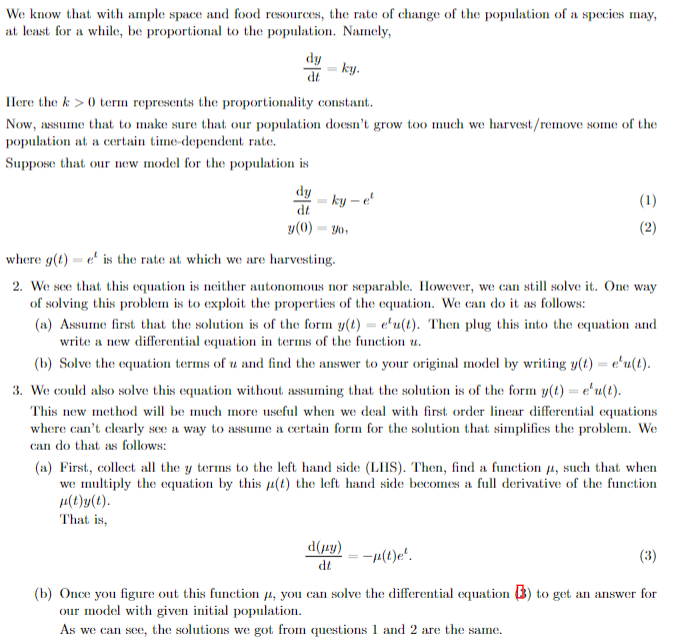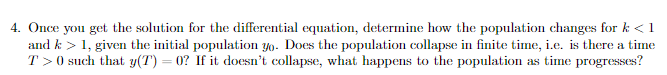# Question Question 4 only. Please explain your work. We know that with ample space and food resources, the rate of change of the population of a species may, at least for a while, be proportional to the population. Namely, $\frac{\mathrm{d} y}{\mathrm{~d} t}=k y$ Here the $$k>0$$ term represents the proportionality constant. Now, assume that to make sure that our population doesn't grow too much we harvest/remove some of the population at a certain time-dependent rate. Suppose that our new model for the population is $\begin{array}{l} \frac{\mathrm{d} y}{\mathrm{~d} t}=k y-e^{t} \\ y(0)=y_{0}, \end{array}$ where $$g(t)=e^{t}$$ is the rate at which we are harvesting. 2. We see that this equation is neither autonomous nor separable. However, we can still solve it. One way of solving this problem is to exploit the properties of the equation. We can do it as follows: (a) Assume first that the solution is of the form $$y(t)=e^{t} u(t)$$. Then plug this into the equation and write a new differential equation in terms of the function $$u$$. (b) Solve the equation terms of $$u$$ and find the answer to your original model by writing $$y(t)=e^{t} u(t)$$. 3. We could also solve this equation without assuming that the solution is of the form $$y(t)=e^{l} u(t)$$. This new method will be much more useful when we deal with first order linear differential equations where can't clearly see a way to assume a certain form for the solution that simplifies the problem. We can do that as follows: (a) First, collect all the $$y$$ terms to the left hand side (LIIS). Then, find a function $$\mu$$, such that when we multiply the equation by this $$\mu(t)$$ the left hand side becomes a full derivative of the function $$\mu(t) y(t)$$ That is, $\frac{\mathrm{d}(\mu y)}{\mathrm{d} t}=-\mu(t) e^{t}$ (b) Once you figure out this function $$\mu$$, you can solve the differential equation (3) to get an answer for our model with given initial population. As we can see, the solutions we got from questions 1 and 2 are the same. 4. Once you get the solution for the differential equation, determine how the population changes for $$k<1$$ and $$k>1$$, given the initial population $$y_{0}$$. Does the population collapse in finite time, i.e. is there a time $$T>0$$ such that $$y(T)=0$$ ? If it doesn't collapse, what happens to the population as time progresses?Transcribed Image Text: We know that with ample space and food resources, the rate of change of the population of a species may, at least for a while, be proportional to the population. Namely, $\frac{\mathrm{d} y}{\mathrm{~d} t}=k y$ Here the $$k>0$$ term represents the proportionality constant. Now, assume that to make sure that our population doesn't grow too much we harvest/remove some of the population at a certain time-dependent rate. Suppose that our new model for the population is $\begin{array}{l} \frac{\mathrm{d} y}{\mathrm{~d} t}=k y-e^{t} \\ y(0)=y_{0}, \end{array}$ where $$g(t)=e^{t}$$ is the rate at which we are harvesting. 2. We see that this equation is neither autonomous nor separable. However, we can still solve it. One way of solving this problem is to exploit the properties of the equation. We can do it as follows: (a) Assume first that the solution is of the form $$y(t)=e^{t} u(t)$$. Then plug this into the equation and write a new differential equation in terms of the function $$u$$. (b) Solve the equation terms of $$u$$ and find the answer to your original model by writing $$y(t)=e^{t} u(t)$$. 3. We could also solve this equation without assuming that the solution is of the form $$y(t)=e^{l} u(t)$$. This new method will be much more useful when we deal with first order linear differential equations where can't clearly see a way to assume a certain form for the solution that simplifies the problem. We can do that as follows: (a) First, collect all the $$y$$ terms to the left hand side (LIIS). Then, find a function $$\mu$$, such that when we multiply the equation by this $$\mu(t)$$ the left hand side becomes a full derivative of the function $$\mu(t) y(t)$$ That is, $\frac{\mathrm{d}(\mu y)}{\mathrm{d} t}=-\mu(t) e^{t}$ (b) Once you figure out this function $$\mu$$, you can solve the differential equation (3) to get an answer for our model with given initial population. As we can see, the solutions we got from questions 1 and 2 are the same. 4. Once you get the solution for the differential equation, determine how the population changes for $$k<1$$ and $$k>1$$, given the initial population $$y_{0}$$. Does the population collapse in finite time, i.e. is there a time $$T>0$$ such that $$y(T)=0$$ ? If it doesn't collapse, what happens to the population as time progresses?
Transcribed Image Text: We know that with ample space and food resources, the rate of change of the population of a species may, at least for a while, be proportional to the population. Namely, $\frac{\mathrm{d} y}{\mathrm{~d} t}=k y$ Here the $$k>0$$ term represents the proportionality constant. Now, assume that to make sure that our population doesn't grow too much we harvest/remove some of the population at a certain time-dependent rate. Suppose that our new model for the population is $\begin{array}{l} \frac{\mathrm{d} y}{\mathrm{~d} t}=k y-e^{t} \\ y(0)=y_{0}, \end{array}$ where $$g(t)=e^{t}$$ is the rate at which we are harvesting. 2. We see that this equation is neither autonomous nor separable. However, we can still solve it. One way of solving this problem is to exploit the properties of the equation. We can do it as follows: (a) Assume first that the solution is of the form $$y(t)=e^{t} u(t)$$. Then plug this into the equation and write a new differential equation in terms of the function $$u$$. (b) Solve the equation terms of $$u$$ and find the answer to your original model by writing $$y(t)=e^{t} u(t)$$. 3. We could also solve this equation without assuming that the solution is of the form $$y(t)=e^{l} u(t)$$. This new method will be much more useful when we deal with first order linear differential equations where can't clearly see a way to assume a certain form for the solution that simplifies the problem. We can do that as follows: (a) First, collect all the $$y$$ terms to the left hand side (LIIS). Then, find a function $$\mu$$, such that when we multiply the equation by this $$\mu(t)$$ the left hand side becomes a full derivative of the function $$\mu(t) y(t)$$ That is, $\frac{\mathrm{d}(\mu y)}{\mathrm{d} t}=-\mu(t) e^{t}$ (b) Once you figure out this function $$\mu$$, you can solve the differential equation (3) to get an answer for our model with given initial population. As we can see, the solutions we got from questions 1 and 2 are the same. 4. Once you get the solution for the differential equation, determine how the population changes for $$k<1$$ and $$k>1$$, given the initial population $$y_{0}$$. Does the population collapse in finite time, i.e. is there a time $$T>0$$ such that $$y(T)=0$$ ? If it doesn't collapse, what happens to the population as time progresses?